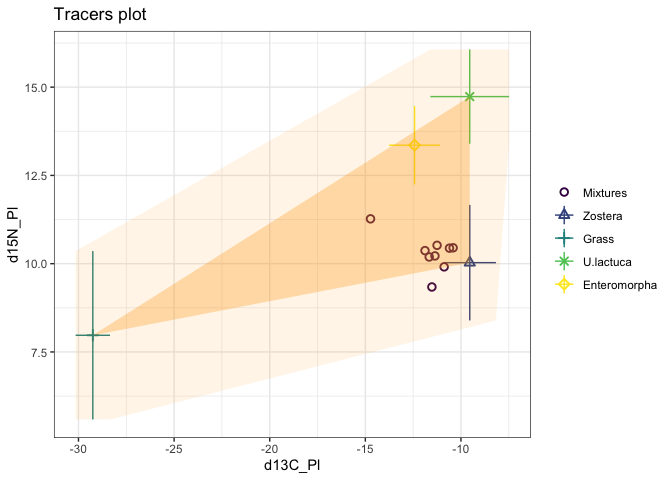## Introduction

Here I am assuming that you have installed simmr and loaded it with:

install.packages("simmr")
library(simmr)

In this vignette are some simple examples of how to customise plots in simmr which shouldn’t require large amounts of additional R knowledge. However…

To customise plots I would recommend having a basic understanding of the ggplot2 package upon which all the simmr graphics are based. A good but long introduction to ggplot2 can be found here: https://ggplot2-book.org. A short version including a cheat sheet is here: https://ggplot2.tidyverse.org. A script which runs through some simple ggplot2 examples is here.

In general, whilst it is possible to customise in a basic way things like axis limits and titles, very detailed customisation of plots is only possible by changing the underlying simmr code. There are different ways to do this, but perhaps the easiest is to download the relevant function from the simmr Github page, and paste it into a new script file. Then you can edit this to produce the required picture.

If you have created other examples of extended simmr plots yourself, please contact me so I can include them here for other people to see.

## A basic simmr run

Consider a basic simmr run (see the other vignettes with vignette(package = 'simmr') for what the commands here mean):

# Load in example data
data(geese_data_day1)
simmr_in <- with(
geese_data_day1,
mixtures = mixtures,
source_names = source_names,
source_means = source_means,
source_sds = source_sds,
correction_means = correction_means,
correction_sds = correction_sds,
concentration_means = concentration_means
)
)
# MCMC run
simmr_out <- simmr_mcmc(simmr_in)

## Customisation of iso-space plots

The iso-space plots in simmr can be customised using arguments in the main body of the function see help(plot.simmr_input) for details. An example would be:

p <- plot(simmr_in,
xlab = expression(paste(delta^13, "C (\u2030)", sep = "")),
ylab = expression(paste(delta^15, "N (\u2030)", sep = "")),
title = "Isospace plot of Inger et al Geese data",
mix_name = "Organism"
)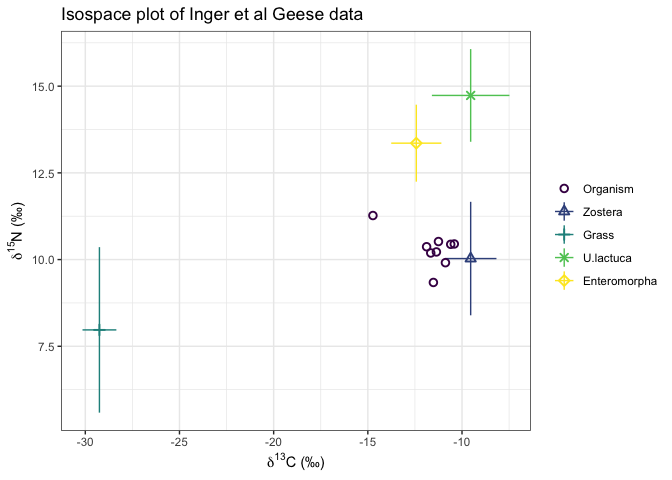You can further customise by adding in extra ggplot commands, for example:

p + xlim(-50, 50) + labs(subtitle = "A subtitle goes here")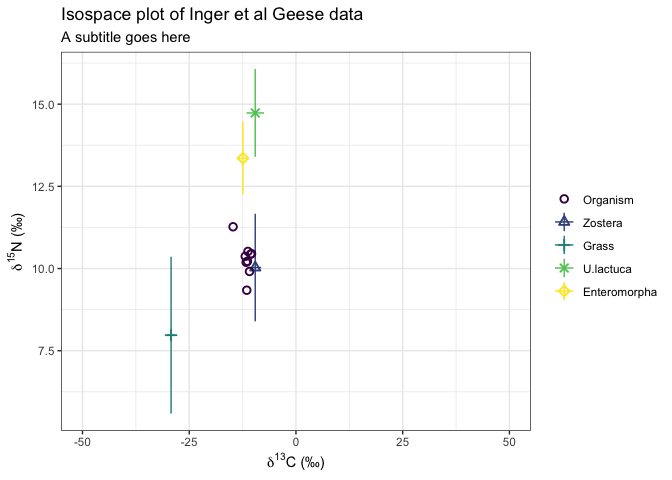## Customisation of output plots

The same idea as above can be used on output plots, for example:

p <- plot(simmr_out,
type = "boxplot",
title = "simmr"
)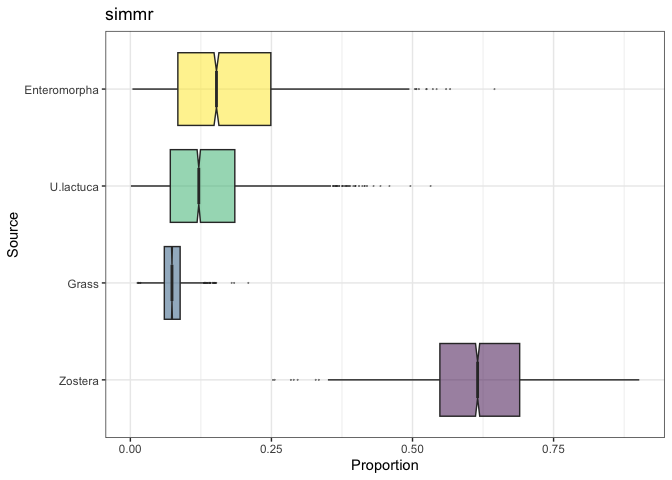Now customise

p + ylim(0, 0.5) +
labs(subtitle = "Something else") +
ylab("A new ylab")
## Warning: Removed 3199 rows containing non-finite values (stat_boxplot()).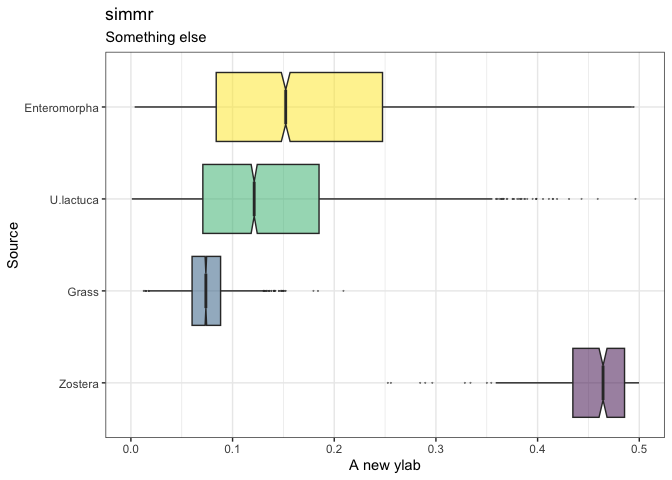If you cut off a part of the graph with e.g. a ylim command then R will provide a warning. Note that the above ylim command actually changes the x-axis and not the y-axis. This is because the coordinates are flipped in the underlying ggplot code which by default uses the source names on the horizontal axis. Here is a more complex example where I change the colours of the boxplots:

# First extract the dietary proportions
simmr_out2 <- simmr_out$output[]$BUGSoutput$sims.list$p
colnames(simmr_out2) <- simmr_out$input$source_names

# Now turn into a proper data frame
library(reshape2)
df <- reshape2::melt(simmr_out2)
colnames(df) <- c("Num", "Source", "Proportion")

# Finally create the new variable that you want to colour by
df$new_colour <- "Type 2" df$new_colour[df$Source == "Zostera"] <- "Type 1" # And create the plot ggplot(df, aes_string( y = "Proportion", x = "Source", fill = "new_colour", alpha = 0, 5 )) + geom_boxplot(notch = TRUE, outlier.size = 0) + theme_bw() + ggtitle("simmr output boxplot with changed colours") + theme(legend.position = "none") + coord_flip() ## Warning: aes_string() was deprecated in ggplot2 3.0.0. ## ℹ Please use tidy evaluation ideoms with aes() ## This warning is displayed once every 8 hours. ## Call lifecycle::last_lifecycle_warnings() to see where this warning was ## generated.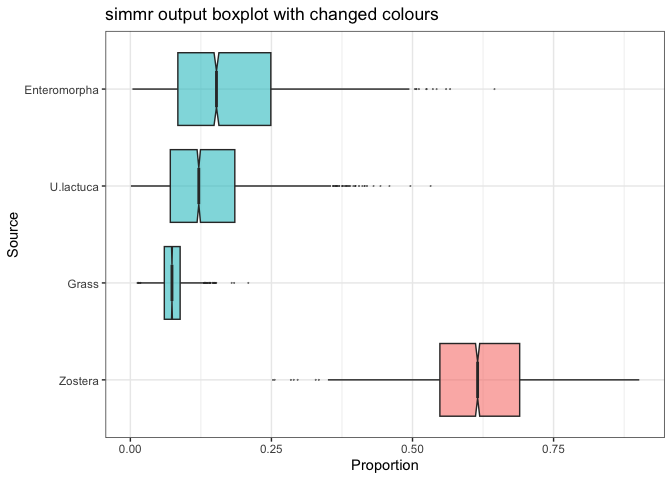## Adding in convex hulls to iso-space plots It’s perfectly possible to add polygons and convex hulls to existing iso-space plots. This does require a bit of hacking though, and is assisted by use of the package ggnewscale. Starting for example with the iso-space plot above: p <- plot(simmr_in)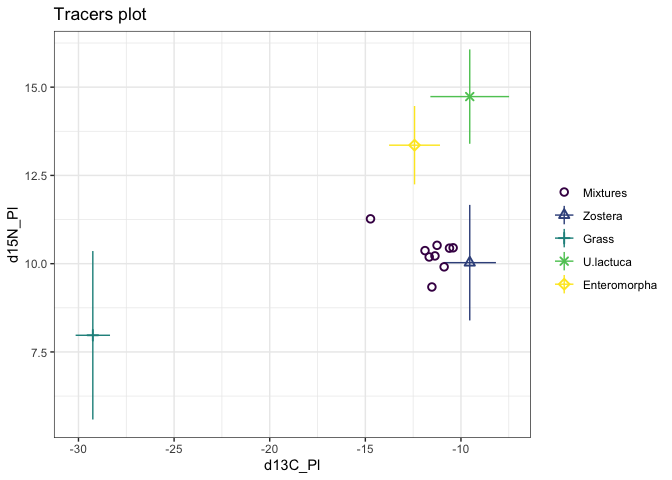We can add a polygon around the mixtures by creating a new data frame with the source mean values (corrected by the TEFs). We have to augment this data frame to include some other parts of the data frame used by the plot.simmr_input function; we can set these to NA. We first create the new data frame. library(ggnewscale) new_df <- data.frame( x = geese_data_day1$source_means[, "meand13CPl"] + geese_data_day1$correction_means[, "meand13CPl"], y = geese_data_day1$source_means[, "meand15NPl"] + geese_data_day1$correction_means[, "meand15NPl"], Source = "Mixtures" ) Then we call the new_scale_color function from ggnewscale with the geom_polygon command to create the polygon p + new_scale_color() + geom_polygon(data = new_df, aes(x = x, y = y), fill = "orange", alpha = 0.2)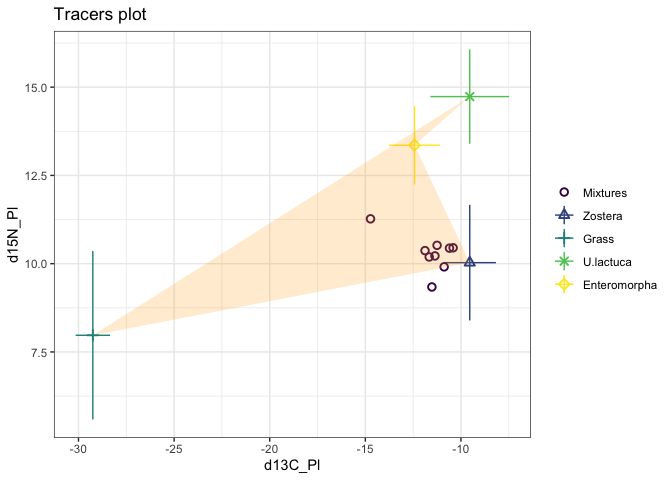If we wanted a convex hull we can use the chull function on the first two columns chull_vals <- chull(new_df[, 1], new_df[, 2]) new_df2 <- new_df[chull_vals, ] p + new_scale_color() + geom_polygon(data = new_df2, aes(x = x, y = y), fill = "orange", alpha = 0.2)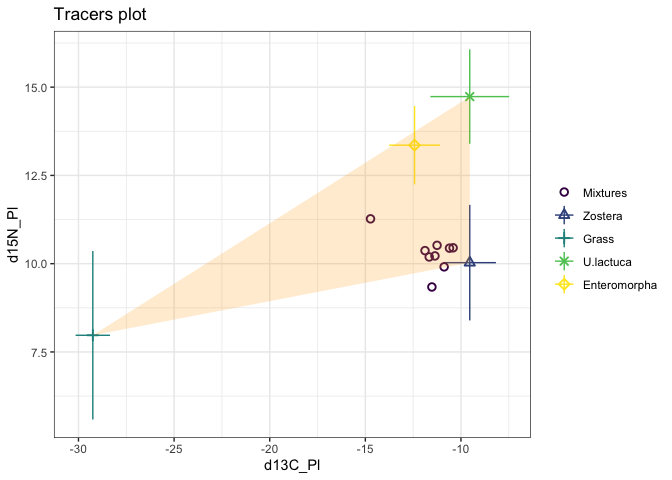Or if we want a convex hull around the mixtures: # Create the new data frame new_mix <- data.frame( x = geese_data_day1$mixtures[, "d13C_Pl"],
y = geese_data_day1$mixtures[, "d15N_Pl"], Source = "Mixtures" ) # Find the convex hull chull_mix_vals <- chull(new_mix[, 1], new_mix[, 2]) new_mix2 <- new_mix[chull_mix_vals, ] # Plot using new_scale_color p + new_scale_color() + geom_polygon(data = new_mix2, aes(x = x, y = y), fill = "purple", alpha = 0.2)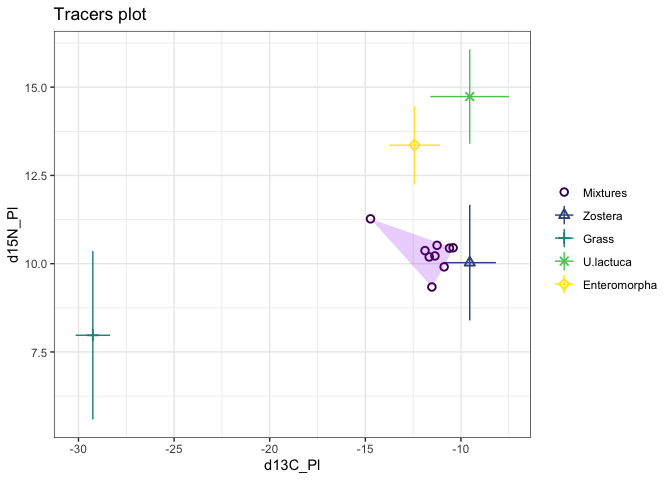We can even do more than 1 convex hull to fully cover e.g. 2 standard deviations of the source area. This might be useful for identifying rogue mixture points. source_means_c <- geese_data_day1$source_means + geese_data_day1$correction_means source_sds_c <- sqrt(geese_data_day1$source_sds^2 + geese_data_day1$correction_sds^2) mix <- geese_data_day1$mixtures
x <- c(
source_means_c[, "meand13CPl"] - source_sds_c[, "SDd13C"],
source_means_c[, "meand13CPl"] - source_sds_c[, "SDd13C"],
source_means_c[, "meand13CPl"] + source_sds_c[, "SDd13C"],
source_means_c[, "meand13CPl"] + source_sds_c[, "SDd13C"]
)
y <- c(
source_means_c[, "meand15NPl"] - source_sds_c[, "SDd15N"],
source_means_c[, "meand15NPl"] + source_sds_c[, "SDd15N"],
source_means_c[, "meand15NPl"] - source_sds_c[, "SDd15N"],
source_means_c[, "meand15NPl"] + source_sds_c[, "SDd15N"]
)
new_df3 <- data.frame(
x = x,
y = y,
Source = "Mixtures"
)
chull_vals <- chull(new_df3[, 1], new_df3[, 2])
new_df4 <- new_df3[chull_vals, ]
p + new_scale_color() +
geom_polygon(data = new_df2, aes(x = x, y = y), fill = "orange", alpha = 0.3) +
new_scale_color() +
geom_polygon(data = new_df4, aes(x = x, y = y), fill = "orange", alpha = 0.1)